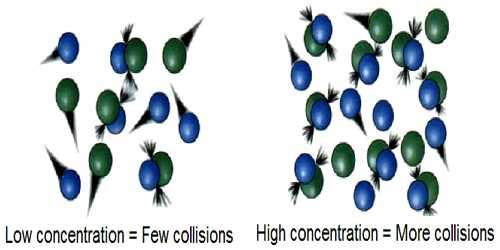# Explain Collision Theory

Collision theory used to forecast the rates of chemical reactions, mainly for gases. This theory is based on the hypothesis that for a reaction to happen it is essential for the reacting species (atoms or molecules) to come together or collide with one another. This theory provides a qualitative explanation of chemical reactions and the rates at which they occur, appealing to the principle that molecules must collide to react.

In all collisions between active molecules lead to reaction the rate of the reaction should be equal to the product of the frequency of collisions and the fraction of molecules which have energies equal to or in excess of Ea. An increase of temperature results in a sharp increase in the number of molecules having energy larger than a given value so that the effects of temperature on reaction rates become understandable. If the total number of collisions per second in a gas at unit concentration is Z, (known as collision frequency), the number of molecules undergoing reaction in unit volume per second, which is equal to the velocity constant k, will be given by –

k = Z e-E/RT … … … (1)

Thus the collision theory leads to a means of calculating reaction rates from molecular collision frequency. Although in a few cases agreement between the calculated and observed values of specific reaction rates has been found, disagreement is more numerous. This suggests that all collisions between active molecules do not lead to reaction. To account for this discrepancy it was necessary to rewrite equation (1) in the form –

k = P Z e-E/RT … … … (2)

Where P is called the steric factor (also called probability factor or geometric factor) and is related to the proper orientation of the active molecules at the time of collision. This factor is independent of temperature changes. The following figures for the reaction between hydrogen and iodine to produce hydrogen iodide will show how the orientation of the molecule at the time of collision may affect the rate of the reaction.

H – H + I – I

As can be seen from the above reaction between H2 and I2, the two reactants must align (orient or line-up) properly for the reaction to take place. However, as the reactant molecules become more complicated, the activated complex becomes more difficult to visualize. Originally P was supposed to measure the geometric requirements that must be met if collisions between two active molecules were to result in reaction. It is possible that many other factors may affect P and it is a measure of the discrepancy between the experimental results and the results predicted by the collision theory. For ordinary thermal reactions the value of P ranges from 10-2 to 10-6 depending on the nature of the reaction.